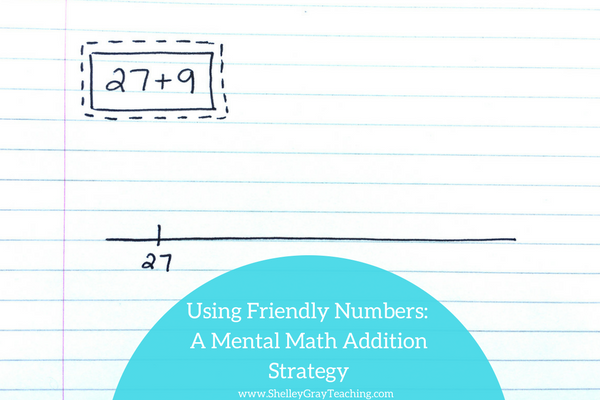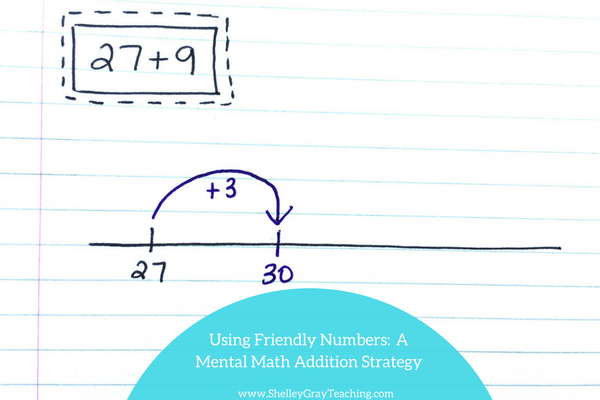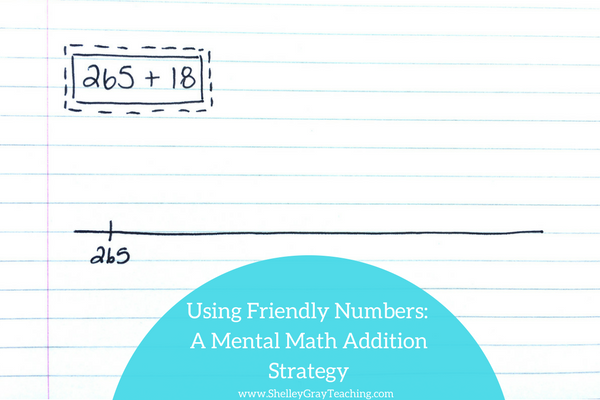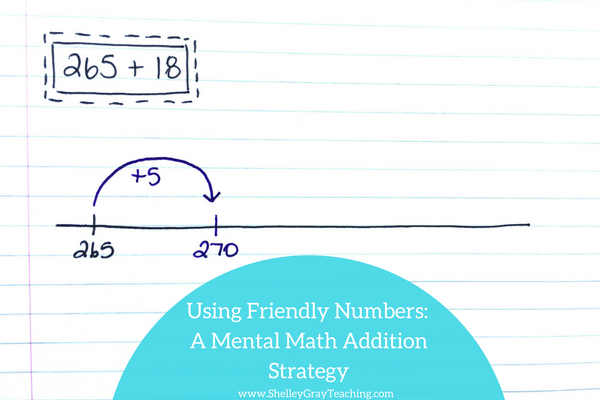# Using Friendly Numbers: An Addition StrategyWHAT IS A FRIENDLY NUMBER?

In this case, we refer to friendly numbers as a number that is easy to work with. For example, multiples of 10 are “friendly” because they are easy to work with when we add or subtract.

USING FRIENDLY NUMBERS AS AN ADDITION STRATEGY

When we use the “friendly number” strategy for addition, it helps us work with big numbers. This is because we are essentially breaking the equation up into more manageable parts.

We begin by getting to a friendly number, which is typically a multiple of 10, 100, or 100 – depending on the numbers that we are working with. Then we add on the remainder.

Let’s take a look at the “using friendly numbers” addition strategy in action.

EXAMPLES

In this example we will add 27+9 using the friendly number strategy.

First, let’s put the number 27 on our empty number line.Now let’s get to a friendly number. We know that the number 30 is “friendly” or easy to work with, so we can add 3 to get to 30.Lastly, we add the remaining 6 and get our answer of 36.Suppose we are solving 265+18.

First we will write 265 on our empty number line.Then we can add 5 from the 18 to get to a friendly number 270.We have 13 left, so now we can simply add the 13 to the 270 to get a final answer of 283.BIG IDEAS

Mental Math is not about following a one size fits all process or procedure. Mental Math involves being able to think flexibly about numbers and manipulate them in different ways.

For the equations shown above, this friendly number strategy worked well. But students also could have used left to right addition, breaking up the an addend, compensation, or even the plus 7,8,9 strategy.

Our goal is to teach our students to think flexibly about numbers so that mental computation comes easily to them.

NEXT STEPS:• Find task cards to reinforce the “using friendly numbers” strategy here:### One Comment

•I LOVE this strategy. I was wondering if you had an anchor chart that I could print and display? Also do you have task cards for 2nd graders? Thank you for your consideration. 🙂